# RD Sharma Solutions for Class 8 Maths Chapter 3 - Squares and Square Roots Exercise 3.4

RD Sharma Solutions for Class 8 Maths Exercise 3.4 Chapter 3, Squares and Square Roots are given below. BYJU’S experts have solved the questions present in Exercise 3.4 in Maths, and this will help students in solving this exercise of Class 8 without any problems. Students who find it challenging to solve tough Math problems can refer to these RD Sharma 8th Maths solutions to get better guidance. Solving this exercise will ensure that students excel in board exams. In this exercise, we shall study the definition of square roots and some properties of square roots. By practising the Solutions of RD Sharma Class 8, students will be able to grasp the concepts entirely, and they can attain good marks in their examinations.

## Download the pdf of RD Sharma Solutions For Class 8 Maths Chapter 3 Squares and Square Roots Exercise 3.4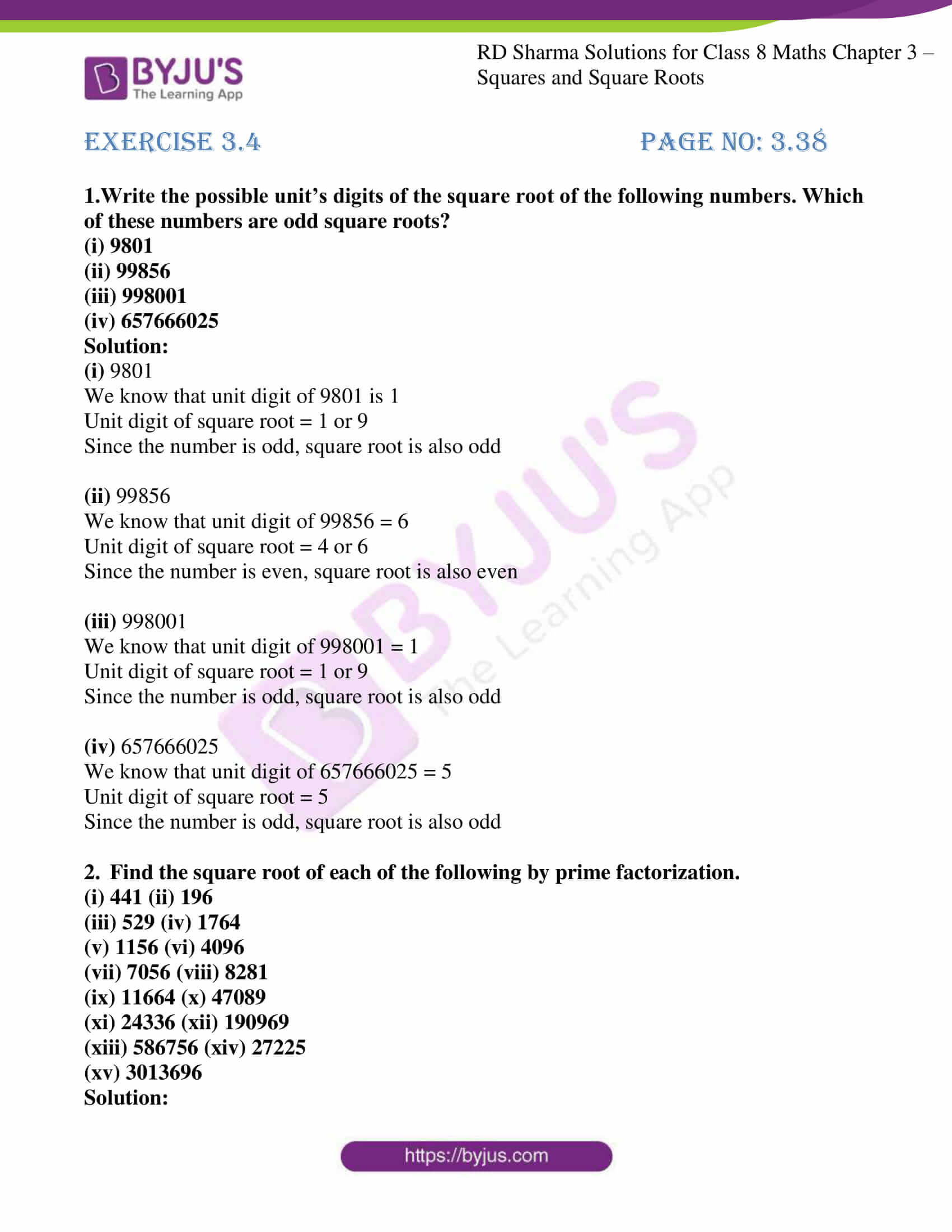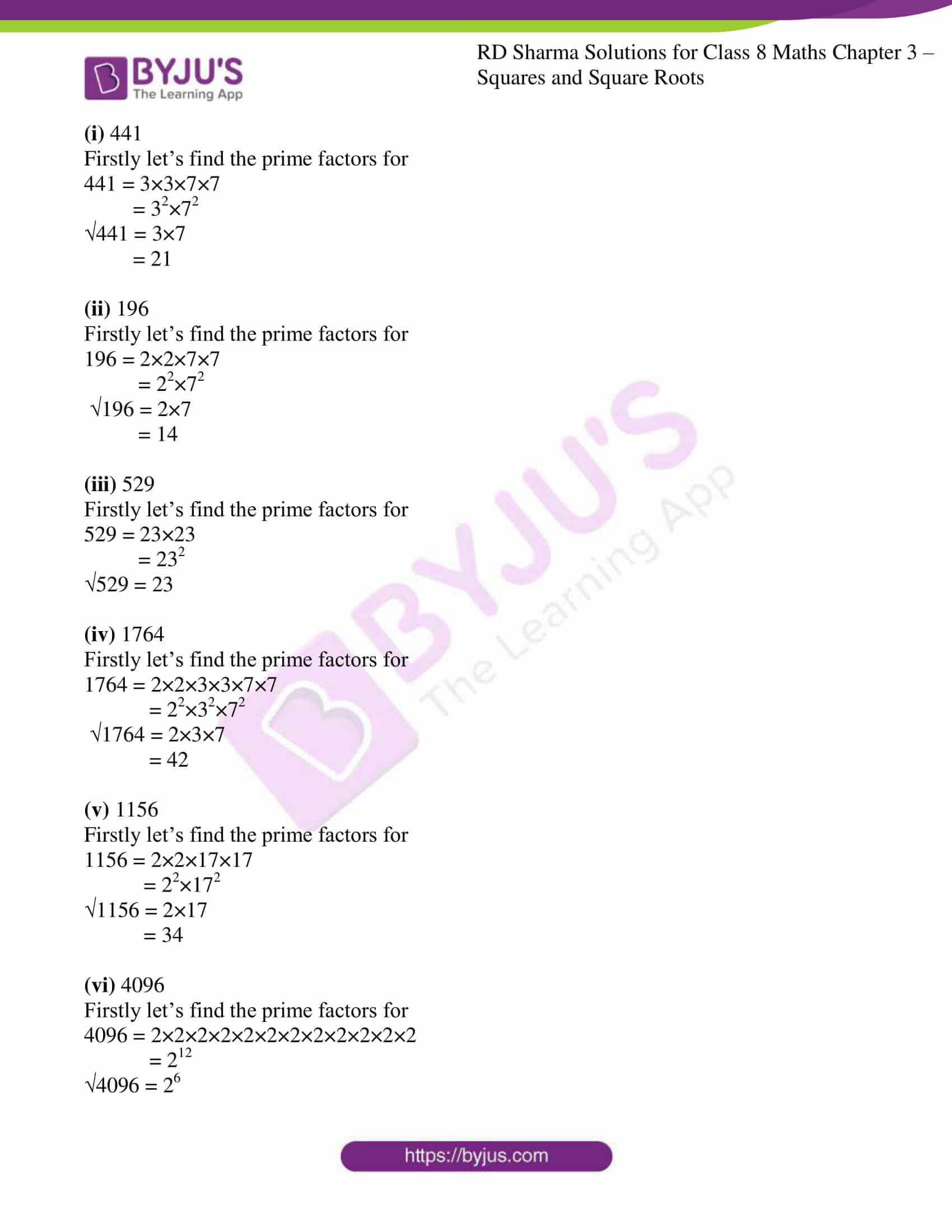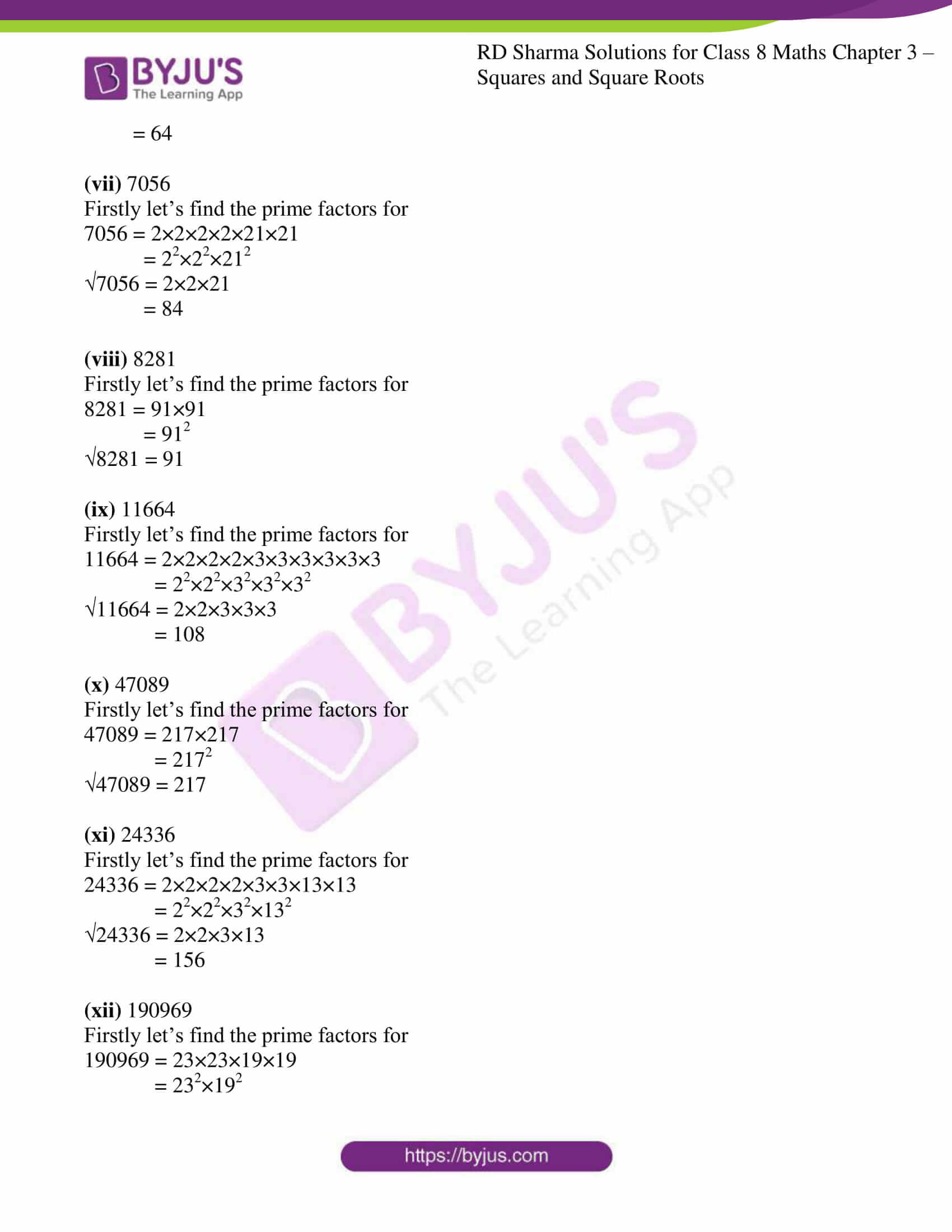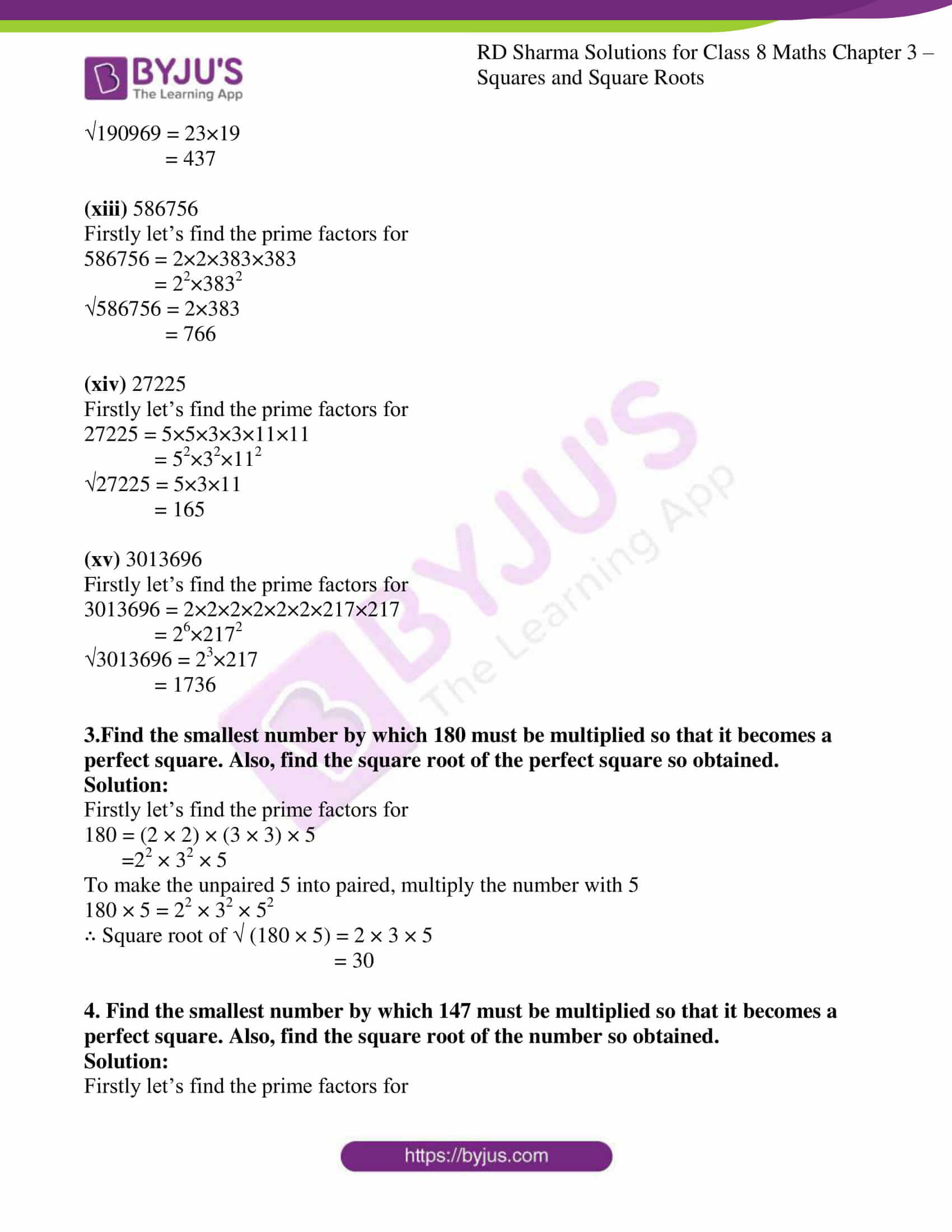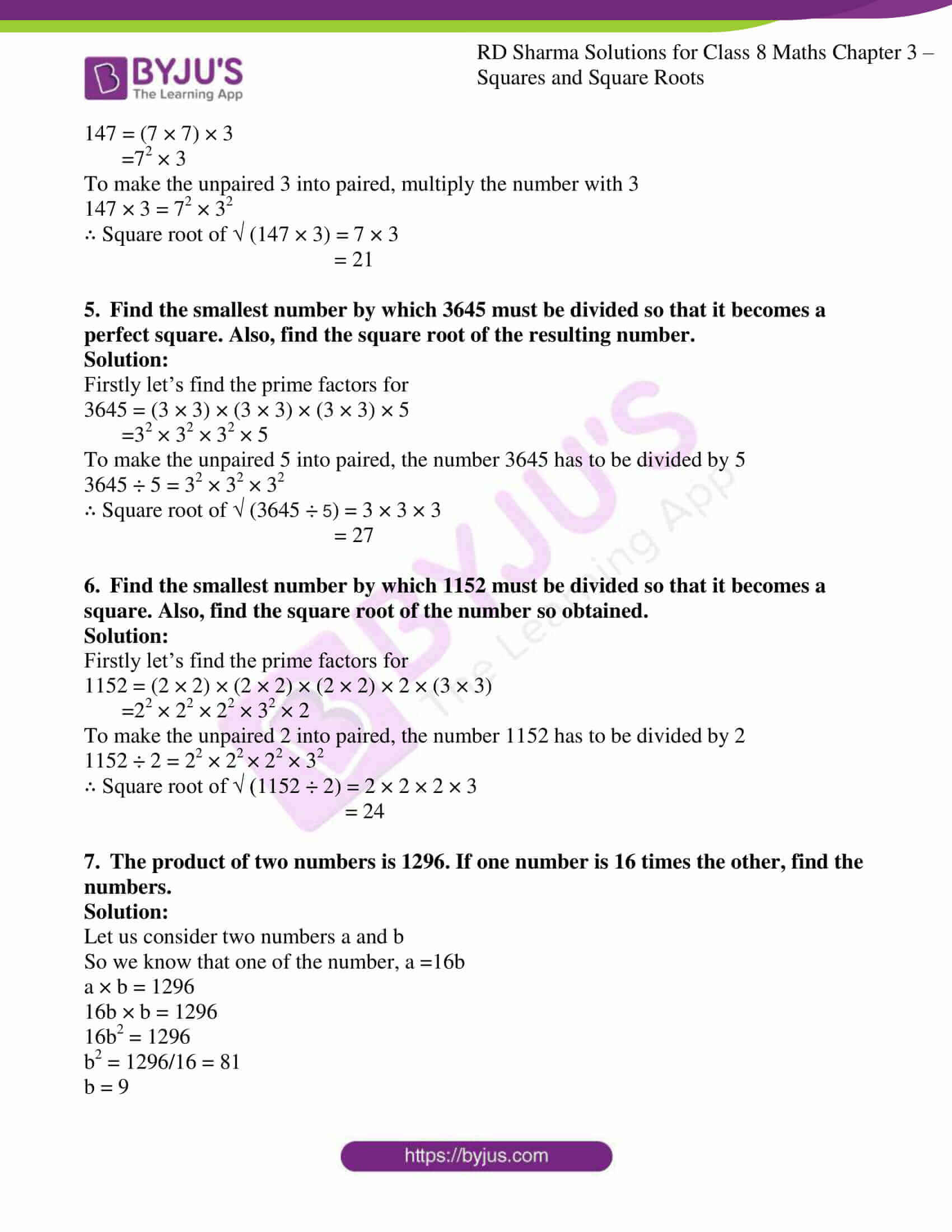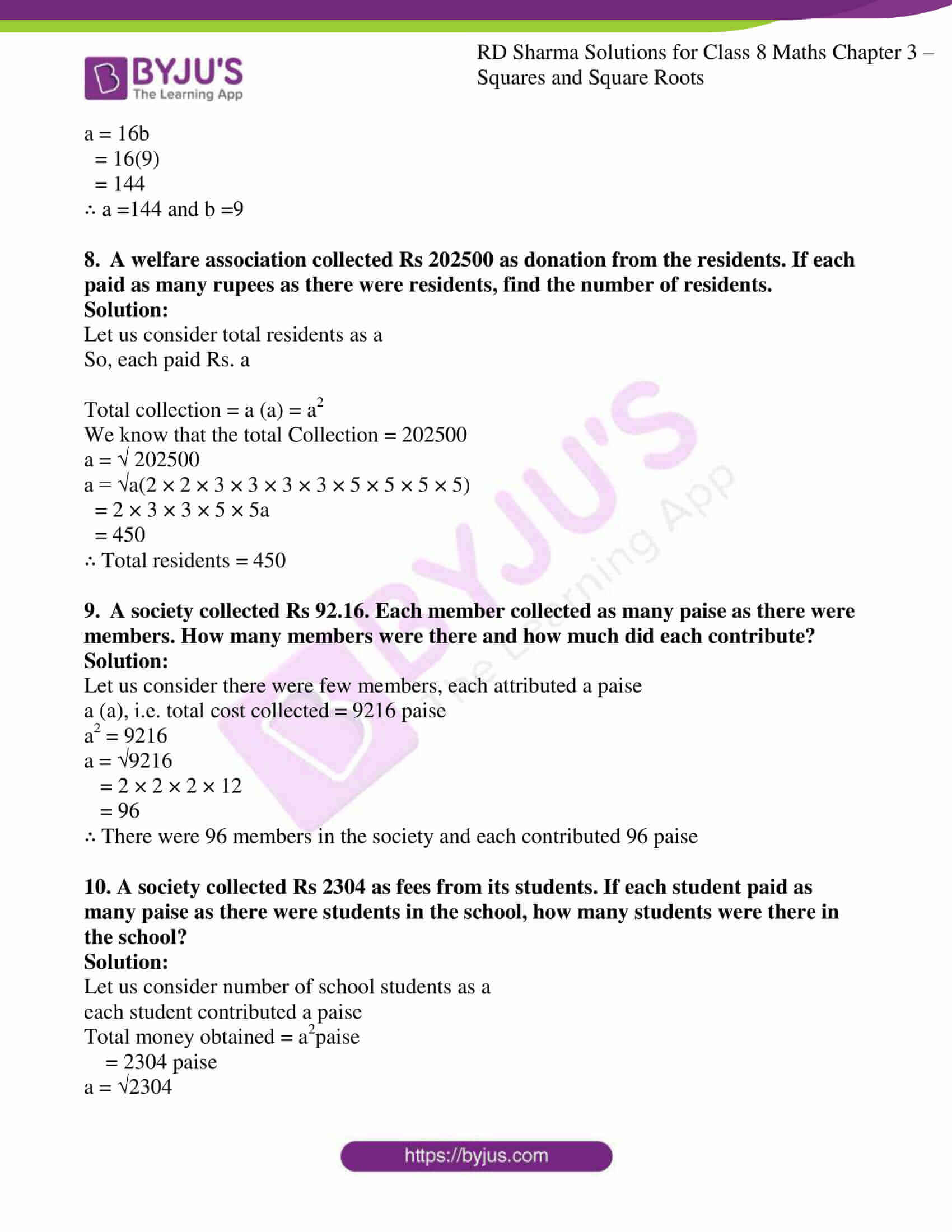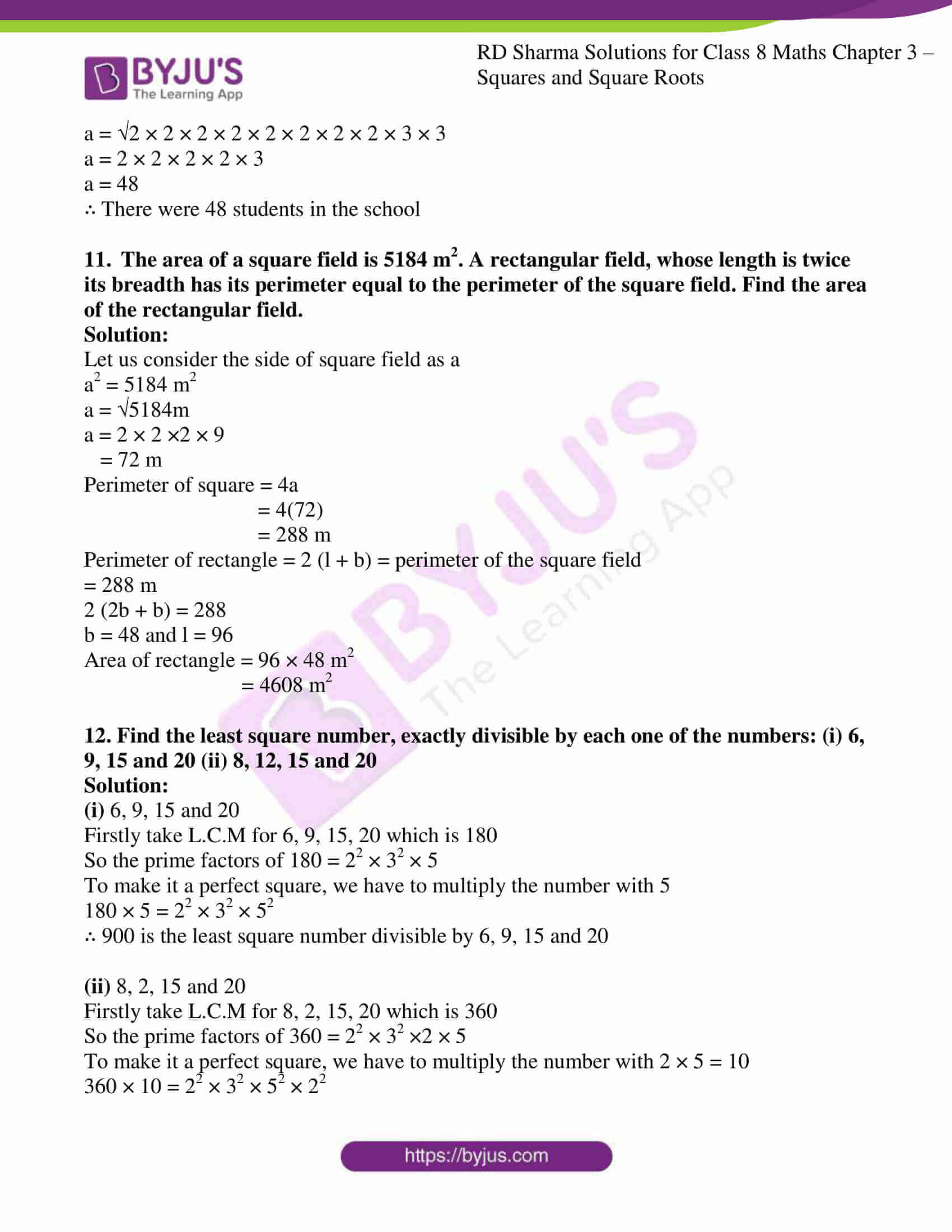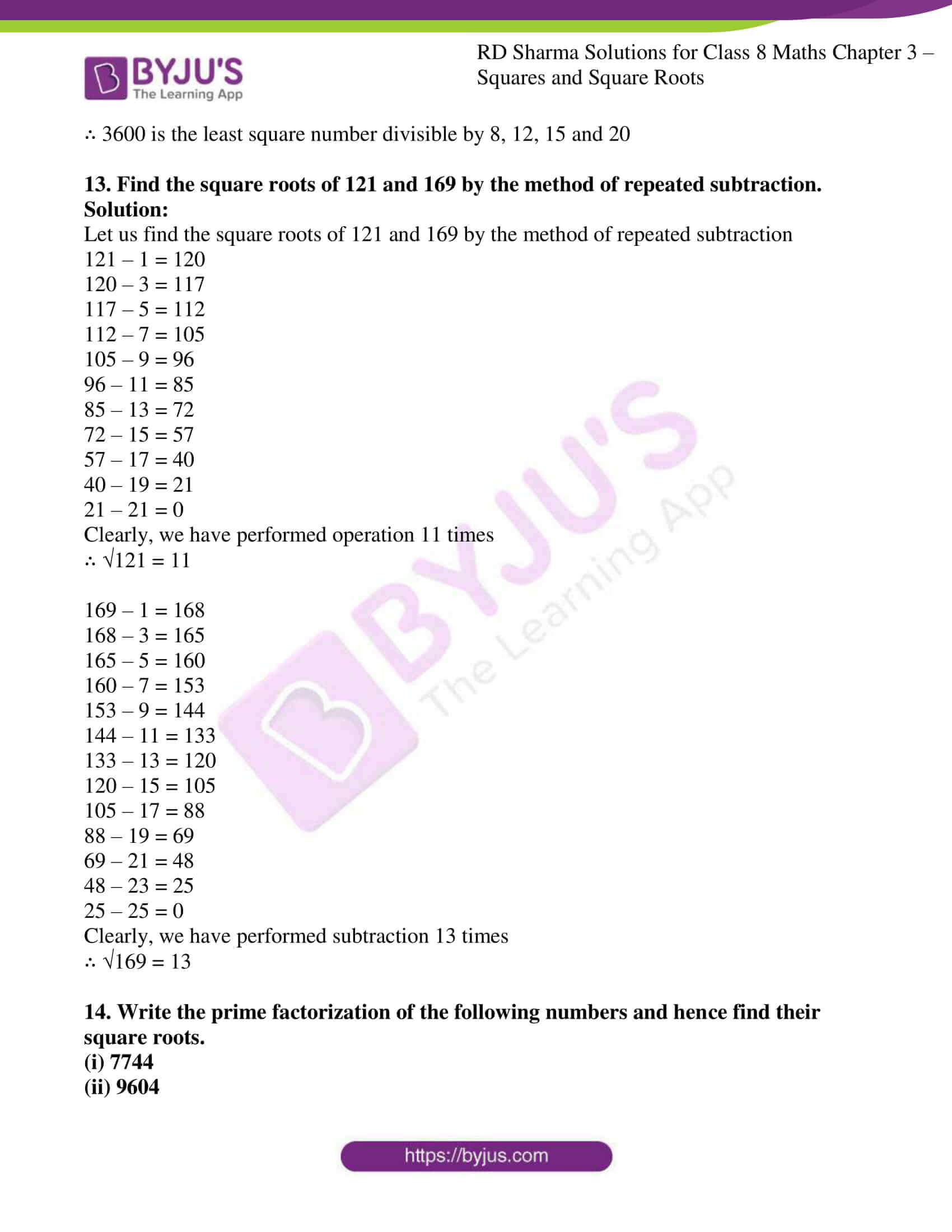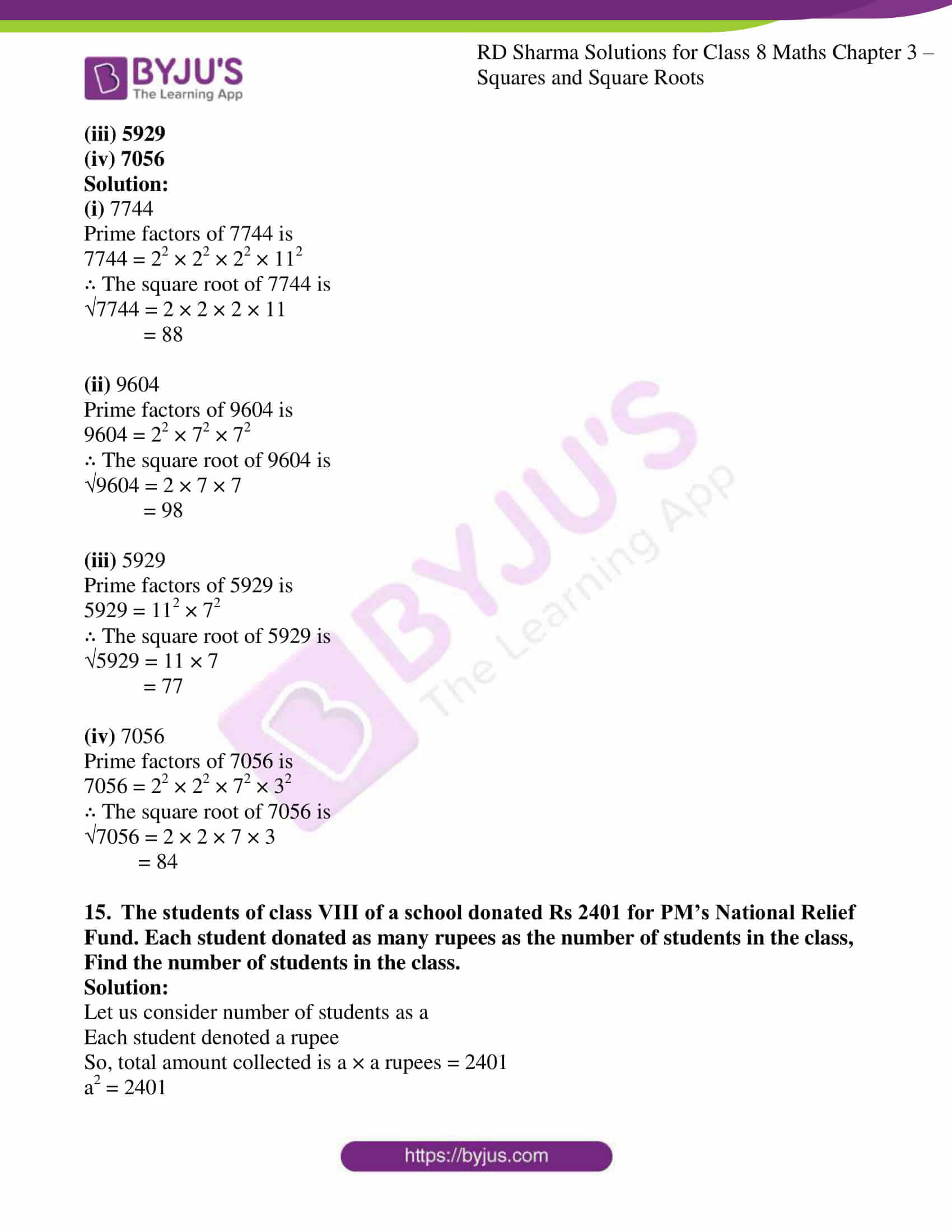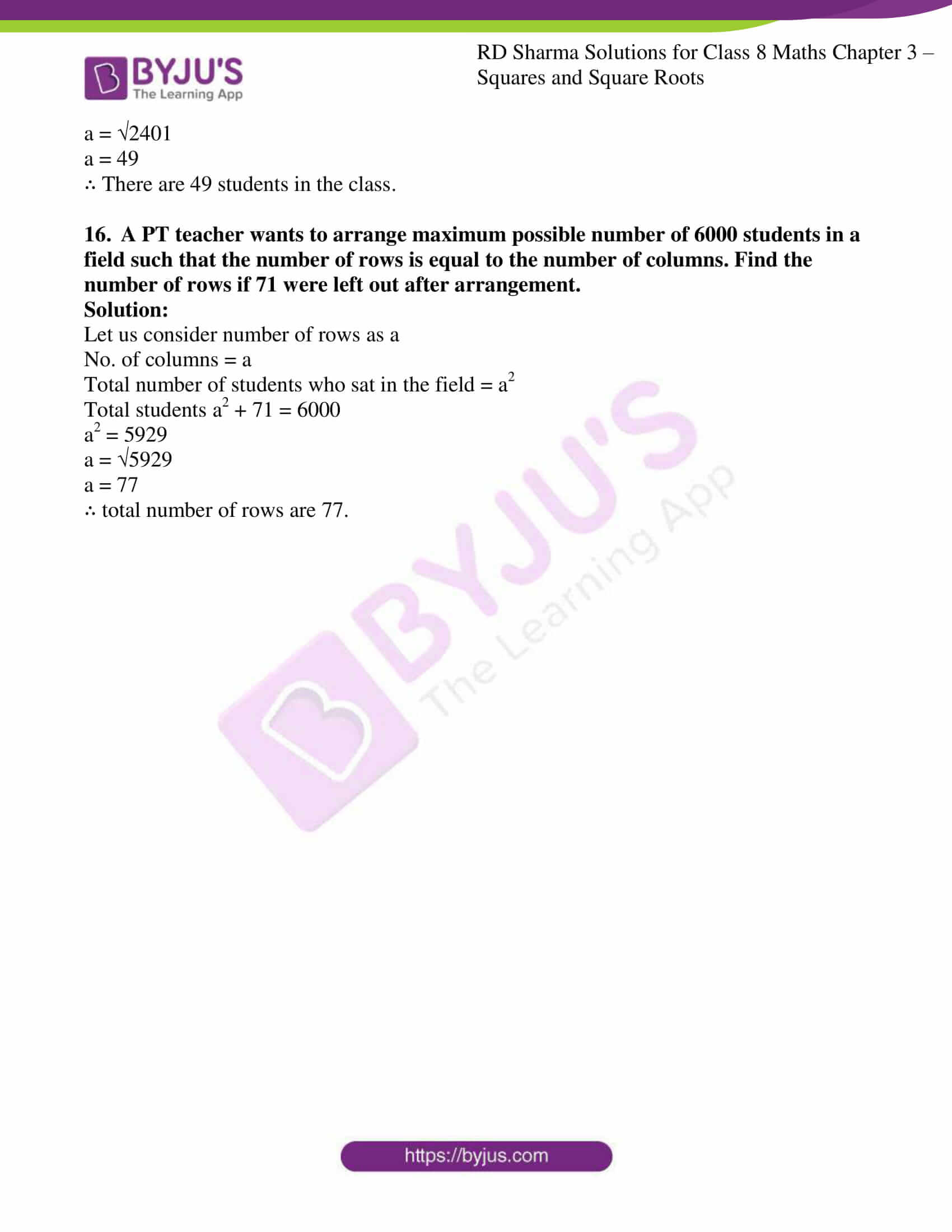### Access Answers to RD Sharma Solutions for Class 8 Maths Exercise 3.4 Chapter 3 Squares and Square Roots

#### EXERCISE 3.4 PAGE NO: 3.38

1.Write the possible unit’s digits of the square root of the following numbers. Which of these numbers are odd square roots?
(i) 9801
(ii) 99856
(iii) 998001
(iv) 657666025

Solution:

(i) 9801

We know that unit digit of 9801 is 1

Unit digit of square root = 1 or 9

Since the number is odd, square root is also odd

(ii) 99856

We know that unit digit of 99856 = 6

Unit digit of square root = 4 or 6

Since the number is even, square root is also even

(iii) 998001

We know that unit digit of 998001 = 1

Unit digit of square root = 1 or 9

Since the number is odd, square root is also odd

(iv) 657666025

We know that unit digit of 657666025 = 5

Unit digit of square root = 5

Since the number is odd, square root is also odd

2. Find the square root of each of the following by prime factorization.
(i) 441 (ii) 196
(iii) 529 (iv) 1764
(v) 1156 (vi) 4096
(vii) 7056 (viii) 8281
(ix) 11664 (x) 47089
(xi) 24336 (xii) 190969
(xiii) 586756 (xiv) 27225
(xv) 3013696

Solution:

(i) 441

Firstly let’s find the prime factors for

441 = 3×3×7×7

= 32×72

√441 = 3×7

= 21

(ii) 196

Firstly let’s find the prime factors for

196 = 2×2×7×7

= 22×72

√196 = 2×7

= 14

(iii)
529

Firstly let’s find the prime factors for

529 = 23×23

= 232

√529 = 23

(iv) 1764

Firstly let’s find the prime factors for

1764 = 2×2×3×3×7×7

= 22×32×72

√1764 = 2×3×7

= 42

(v)
1156

Firstly let’s find the prime factors for

1156 = 2×2×17×17

= 22×172

√1156 = 2×17

= 34

(vi) 4096

Firstly let’s find the prime factors for

4096 = 2×2×2×2×2×2×2×2×2×2×2×2

= 212

√4096 = 26

= 64

(vii) 7056

Firstly let’s find the prime factors for

7056 = 2×2×2×2×21×21

= 22×22×212

√7056 = 2×2×21

= 84

(viii) 8281

Firstly let’s find the prime factors for

8281 = 91×91

= 912

√8281 = 91

(ix)
11664

Firstly let’s find the prime factors for

11664 = 2×2×2×2×3×3×3×3×3×3

= 22×22×32×32×32

√11664 = 2×2×3×3×3

= 108

(x) 47089

Firstly let’s find the prime factors for

47089 = 217×217

= 2172

√47089 = 217

(xi)
24336

Firstly let’s find the prime factors for

24336 = 2×2×2×2×3×3×13×13

= 22×22×32×132

√24336 = 2×2×3×13

= 156

(xii) 190969

Firstly let’s find the prime factors for

190969 = 23×23×19×19

= 232×192

√190969 = 23×19

= 437

(xiii)
586756

Firstly let’s find the prime factors for

586756 = 2×2×383×383

= 22×3832

√586756 = 2×383

= 766

(xiv) 27225

Firstly let’s find the prime factors for

27225 = 5×5×3×3×11×11

= 52×32×112

√27225 = 5×3×11

= 165

(xv)
3013696

Firstly let’s find the prime factors for

3013696 = 2×2×2×2×2×2×217×217

= 26×2172

√3013696 = 23×217

= 1736

3.Find the smallest number by which 180 must be multiplied so that it becomes a perfect square. Also, find the square root of the perfect square so obtained.

Solution:

Firstly let’s find the prime factors for

180 = (2 × 2) × (3 × 3) × 5

=22 × 32 × 5

To make the unpaired 5 into paired, multiply the number with 5

180 × 5 = 22 × 32 × 52

∴ Square root of √ (180 × 5) = 2 × 3 × 5

= 30

4. Find the smallest number by which 147 must be multiplied so that it becomes a perfect square. Also, find the square root of the number so obtained.

Solution:

Firstly let’s find the prime factors for

147 = (7 × 7) × 3

=72 × 3

To make the unpaired 3 into paired, multiply the number with 3

147 × 3 = 72 × 32

∴ Square root of √ (147 × 3) = 7 × 3

= 21

5. Find the smallest number by which 3645 must be divided so that it becomes a perfect square. Also, find the square root of the resulting number.

Solution:

Firstly let’s find the prime factors for

3645 = (3 × 3) × (3 × 3) × (3 × 3) × 5

=32 × 32 × 32 × 5

To make the unpaired 5 into paired, the number 3645 has to be divided by 5

3645 ÷ 5 = 32 × 32 × 32

∴ Square root of √ (3645 ÷ 5) = 3 × 3 × 3

= 27

6. Find the smallest number by which 1152 must be divided so that it becomes a square. Also, find the square root of the number so obtained.

Solution:

Firstly let’s find the prime factors for

1152 = (2 × 2) × (2 × 2) × (2 × 2) × 2 × (3 × 3)

=22 × 22 × 22 × 32 × 2

To make the unpaired 2 into paired, the number 1152 has to be divided by 2

1152 ÷ 2 = 22 × 22 × 22 × 32

∴ Square root of √ (1152 ÷ 2) = 2 × 2 × 2 × 3

= 24

7. The product of two numbers is 1296. If one number is 16 times the other, find the numbers.

Solution:

Let us consider two numbers a and b

So we know that one of the number, a =16b

a × b = 1296

16b × b = 1296

16b2 = 1296

b2 = 1296/16 = 81

b = 9

a = 16b

= 16(9)

= 144

∴ a =144 and b =9

8. A welfare association collected Rs 202500 as donation from the residents. If each paid as many rupees as there were residents, find the number of residents.

Solution:

Let us consider total residents as a

So, each paid Rs. a

Total collection = a (a) = a2

We know that the total Collection = 202500

a = √ 202500

a = √a(2 × 2 × 3 × 3 × 3 × 3 × 5 × 5 × 5 × 5)

= 2 × 3 × 3 × 5 × 5a

= 450

∴ Total residents = 450

9. A society collected Rs 92.16. Each member collected as many paise as there were members. How many members were there and how much did each contribute?

Solution:

Let us consider there were few members, each attributed a paise

a (a), i.e. total cost collected = 9216 paise

a2 = 9216

a = √9216

= 2 × 2 × 2 × 12

= 96

∴ There were 96 members in the society and each contributed 96 paise

10. A society collected Rs 2304 as fees from its students. If each student paid as many paise as there were students in the school, how many students were there in the school?

Solution:

Let us consider number of school students as a

each student contributed a paise

Total money obtained = a2paise

= 2304 paise

a = √2304

a = √2 × 2 × 2 × 2 × 2 × 2 × 2 × 2 × 3 × 3

a = 2 × 2 × 2 × 2 × 3

a = 48

∴ There were 48 students in the school

11. The area of a square field is 5184 m2. A rectangular field, whose length is twice its breadth has its perimeter equal to the perimeter of the square field. Find the area of the rectangular field.

Solution:

Let us consider the side of square field as a

a2 = 5184 m2

a = √5184m

a = 2 × 2 ×2 × 9

= 72 m

Perimeter of square = 4a

= 4(72)

= 288 m

Perimeter of rectangle = 2 (l + b) = perimeter of the square field

= 288 m

2 (2b + b) = 288

b = 48 and l = 96

Area of rectangle = 96 × 48 m2

= 4608 m2

12. Find the least square number, exactly divisible by each one of the numbers: (i) 6, 9, 15 and 20 (ii) 8, 12, 15 and 20

Solution:

(i) 6, 9, 15 and 20

Firstly take L.C.M for 6, 9, 15, 20 which is 180

So the prime factors of 180 = 22 × 32 × 5

To make it a perfect square, we have to multiply the number with 5

180 × 5 = 22 × 32 × 52

∴ 900 is the least square number divisible by 6, 9, 15 and 20

(ii) 8, 2, 15 and 20

Firstly take L.C.M for 8, 2, 15, 20 which is 360

So the prime factors of 360 = 22 × 32 ×2 × 5

To make it a perfect square, we have to multiply the number with 2 × 5 = 10

360 × 10 = 22 × 32 × 52 × 22

∴ 3600 is the least square number divisible by 8, 12, 15 and 20

13. Find the square roots of 121 and 169 by the method of repeated subtraction.

Solution:

Let us find the square roots of 121 and 169 by the method of repeated subtraction

121 – 1 = 120

120 – 3 = 117

117 – 5 = 112

112 – 7 = 105

105 – 9 = 96

96 – 11 = 85

85 – 13 = 72

72 – 15 = 57

57 – 17 = 40

40 – 19 = 21

21 – 21 = 0

Clearly, we have performed operation 11 times

∴ √121 = 11

169 – 1 = 168

168 – 3 = 165

165 – 5 = 160

160 – 7 = 153

153 – 9 = 144

144 – 11 = 133

133 – 13 = 120

120 – 15 = 105

105 – 17 = 88

88 – 19 = 69

69 – 21 = 48

48 – 23 = 25

25 – 25 = 0

Clearly, we have performed subtraction 13 times

∴ √169 = 13

14. Write the prime factorization of the following numbers and hence find their square roots.
(i) 7744

(ii) 9604
(iii) 5929
(iv) 7056

Solution:

(i) 7744

Prime factors of 7744 is

7744 = 22 × 22 × 22 × 112

∴ The square root of 7744 is

√7744 = 2 × 2 × 2 × 11

= 88

(ii) 9604

Prime factors of 9604 is

9604 = 22 × 72 × 72

∴ The square root of 9604 is

√9604 = 2 × 7 × 7

= 98

(iii) 5929

Prime factors of 5929 is

5929 = 112 × 72

∴ The square root of 5929 is

√5929 = 11 × 7

= 77

(iv) 7056

Prime factors of 7056 is

7056 = 22 × 22 × 72 × 32

∴ The square root of 7056 is

√7056 = 2 × 2 × 7 × 3

= 84

15. The students of class VIII of a school donated Rs 2401 for PM’s National Relief Fund. Each student donated as many rupees as the number of students in the class, Find the number of students in the class.

Solution:

Let us consider number of students as a

Each student denoted a rupee

So, total amount collected is a × a rupees = 2401

a2 = 2401

a = √2401

a = 49

∴ There are 49 students in the class.

16. A PT teacher wants to arrange maximum possible number of 6000 students in a field such that the number of rows is equal to the number of columns. Find the number of rows if 71 were left out after arrangement.

Solution:

Let us consider number of rows as a

No. of columns = a

Total number of students who sat in the field = a2

Total students a2 + 71 = 6000

a2 = 5929

a = √5929

a = 77

∴ total number of rows are 77.

## RD Sharma Solutions for Class 8 Maths Exercise 3.4 Chapter 3 – Squares and Square Roots

Exercise 3.4 of RD Sharma Solutions for Chapter 3 Squares and Square Roots, this exercise mainly deals with the definition and methods to find the square root of a perfect square by the prime factorisation method. The RD Sharma Solutions can help the students practice diligently while learning the fundamentals as it provides all the answers to the questions from the RD Sharma textbook. Students are suggested to practice the problems regularly which will help them ace their exams and increase their overall percentage.